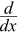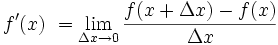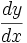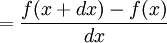# Introduction to Derivatives

 Slope = Change in YChange in XWe can find an average slope between two points.But how do we find the slope at a point? There is nothing to measure!But with derivatives we use a small difference ... ... then have it shrink towards zero.## Let us Find a Derivative!

To find the derivative of a function y = f(x) we use the slope formula:

Slope = Change in Y Change in X = ΔyΔxAnd (from the diagram) we see that:

 x changes from x to x+Δx y changes from f(x) to f(x+Δx)

• Fill in this slope formula: ΔyΔx = f(x+Δx) − f(x)Δx
• Simplify it as best we can
• Then make Δx shrink towards zero.

Like this:

### Example: the function f(x) = x2

We know f(x) = x2, and we can calculate f(x+Δx) :

 Start with: f(x+Δx) = (x+Δx)2 Expand (x + Δx)2: f(x+Δx) = x2 + 2x Δx + (Δx)2

The slope formula is: f(x+Δx) − f(x) Δx
Put in f(x+Δx) and f(x): x2 + 2x Δx + (Δx)2 − x2 Δx
Simplify (x2 and −x2 cancel): 2x Δx + (Δx)2 Δx
Simplify more (divide through by Δx):= 2x + Δx
Then as Δx heads towards 0 we get:= 2x

Result: the derivative of x2 is 2x

In other words, the slope at x is 2x

And "the derivative of" is commonly written:x2 = 2x
"The derivative of x2 equals 2x"
or simply "d dx of x2 equals 2x"### What doesx2 = 2x mean?

It means that, for the function x2, the slope or "rate of change" at any point is 2x.

So when x=2 the slope is 2x = 4, as shown here:

Or when x=5 the slope is 2x = 10, and so on.

Note: sometimes f’(x) is also used for "the derivative of":

f’(x) = 2x
"The derivative of f(x) equals 2x"
or simply "f-dash of x equals 2x"

Let's try another example.

### Example: What isx3 ?

We know f(x) = x3, and can calculate f(x+Δx) :

 Start with: f(x+Δx) = (x+Δx)3 Expand (x + Δx)3: f(x+Δx) = x3 + 3x2 Δx + 3x (Δx)2 + (Δx)3

The slope formula: f(x+Δx) − f(x) Δx
Put in f(x+Δx) and f(x): x3 + 3x2 Δx + 3x (Δx)2 + (Δx)3 − x3 Δx
Simplify (x3 and −x3 cancel): 3x2 Δx + 3x (Δx)2 + (Δx)3 Δx
Simplify more (divide through by Δx): = 3x2 + 3x Δx + (Δx)2
Then as Δx heads towards 0 we get:= 3x2

Result: the derivative of x3 is 3x2

Have a play with it using the Derivative Plotter.

## Derivatives of Other Functions

We can use the same method to work out derivatives of other functions (like sine, cosine, logarithms, etc).

But in practice the usual way to find derivatives is to use:

Derivative Rules

### Example: what is the derivative of sin(x) ?

On Derivative Rules it is listed as being cos(x)

Done.

Using the rules can be tricky!

### Example: what is the derivative of cos(x)sin(x) ?

You can't just find the derivative of cos(x) and multiply it by the derivative of sin(x) ... you must use the "Product Rule" as explained on the Derivative Rules page.

It actually works out to be cos2(x) − sin2(x)

So that is your next step: learn how to use the rules.

## Notation

"Shrink towards zero" is actually written as a limit like this:"The derivative of f equals the limit as Δx goes to zero of f(x+Δx) - f(x) over Δx"

Or sometimes the derivative is written like this (explained on Derivatives as dy/dx):The process of finding a derivative is called "differentiation".

You do differentiation ... to get a derivative.

## Where to Next?

Go and learn how to find derivatives using Derivative Rules, and get plenty of practice: